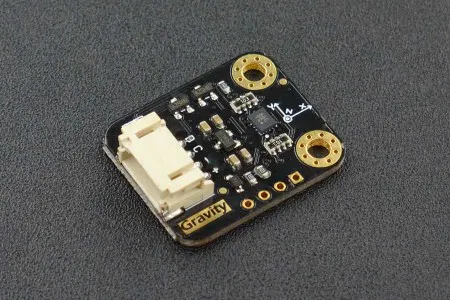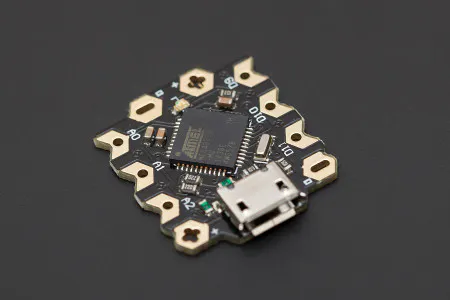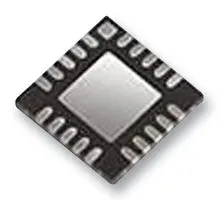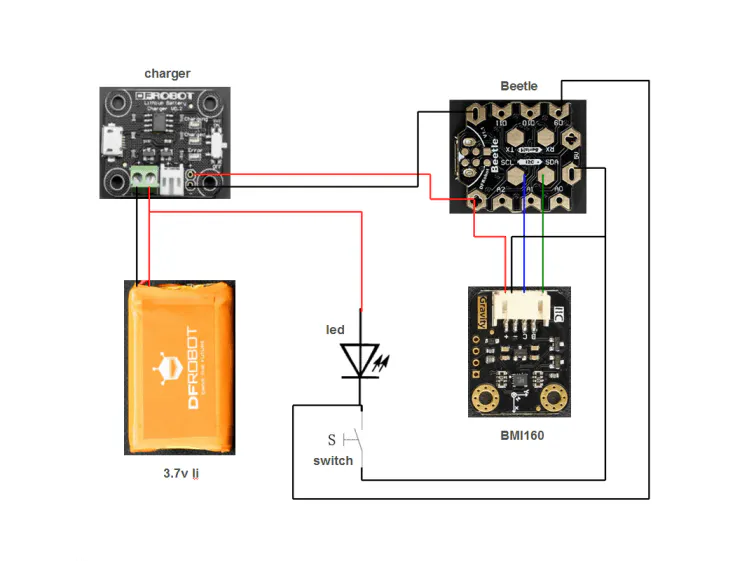# How to Make a Simple Digital Globe Lamp

I want to turn on the light simply by lightly knocking on the table.

IntermediateFull instructions provided6 hours540## Things used in this project

### Hardware componentsDFRobot Gravity: I2C BMI160 6-Axis Inertial Motion Sensor
×1DFRobot Beetle - The Smallest Arduino
×1Battery Charger, 1 Cell Lithium Battery
×1
×10

### Hand tools and fabrication machines

 A small amount of acrylic pigment (green, yellow, blue and brown; if gray and white pigment is provided, the oil paint does not need to be prepared)
 Light gray and white oil paint
 A good-looking 2-foot button (I bought it on Taobao)Lead wire (2 mm) (If no, replace it with soft iron wire)
 40 cm PVC hollow rod (I use the one with outer diameter 9 mm and inner diameter 5 mm)
 A screw and nut with the length of 20mm

## Schematics

### Connection Diagram## Code

### sketch_oct18a

C/C++
```#include "DFRobot_BMI160.h"

DFRobot_BMI160 bmi160;
int ledPin=9;
float Gyro_abs={{0,0,0,0,0,0,0,0,0,0,0,0,0,0,0,0,0,0,0,0,0,0,0,0,0,0,0,0,0,0,0,0,0,0,0},
{0,0,0,0,0,0,0,0,0,0,0,0,0,0,0,0,0,0,0,0,0,0,0,0,0,0,0,0,0,0,0,0,0,0,0},
{0,0,0,0,0,0,0,0,0,0,0,0,0,0,0,0,0,0,0,0,0,0,0,0,0,0,0,0,0,0,0,0,0,0,0}};//accelX/Y/Z,GyroX/Y/Z
float Gyro_buf={{0,0,0,0,0,0,0,0,0,0,0,0,0,0,0,0,0,0,0,0,0,0,0,0,0,0,0,0,0,0,0,0,0,0,0},
{0,0,0,0,0,0,0,0,0,0,0,0,0,0,0,0,0,0,0,0,0,0,0,0,0,0,0,0,0,0,0,0,0,0,0},
{0,0,0,0,0,0,0,0,0,0,0,0,0,0,0,0,0,0,0,0,0,0,0,0,0,0,0,0,0,0,0,0,0,0,0}};
float accel_abs={{0,0,0,0,0},
{0,0,0,0,0},
{0,0,0,0,0}};
float aG_step={0,0,0,0,0,0};
float aG_now={0,0,0,0,0,0};
float aG_last={0,0,0,0,0,0};
float aG_ave={0,0,0,0,0,0};
boolean shakeFlag=0;
boolean singleFlag=0;
boolean doubleFlag=0;
boolean doubleState=0;
boolean count_begin=0;
boolean noState=1;
boolean ledState=1;
byte count_times=0;
byte state=0b00000000;

void setup(){
Serial.begin(115200);
pinMode(ledPin,OUTPUT);
digitalWrite(ledPin,LOW);
delay(100);

//init the hardware bmin160
if (bmi160.softReset() != BMI160_OK){
Serial.println("reset false");
while(1);
}

//set and init the bmi160 i2c address
Serial.println("init false");
while(1);
}
}

{
int i = 0;
int rslt;
int16_t accelGyro={0};
float dat={0};
//get both accel and gyro data from bmi160
//parameter accelGyro is the pointer to store the data
rslt = bmi160.getAccelGyroData(accelGyro);
if(rslt == 0){
for(i=0;i<6;i++){
if (i<3){
//the first three are gyro datas
dat[i]=accelGyro[i]*3.14/180.0;
Serial.print(dat[i]);Serial.print("\t");
}else{
//the following three data are accel datas
dat[i]=accelGyro[i]/16384.0;
Serial.print(dat[i]);Serial.print("\t");
}
}
Serial.println();
}else{
Serial.println("err");
}
return dat;
}

{
byte x,y,z;
//Serial.print("step:");
for(x=0;x<6;x++)
{
aG_step[x]=aG_now[x]-aG_last[x];
aG_step[x]=abs(aG_step[x]);
//Serial.print(aG_step[x]);
//Serial.print("\t");
aG_last[x]=aG_now[x];
}
//Serial.println();
for(x=0;x<3;x++)
{
for(y=0;y<34;y++)
{
Gyro_abs[x][y]=Gyro_abs[x][y+1];
Gyro_buf[x][y]=Gyro_abs[x][y+1];
}
}
for(x=0;x<3;x++)
{
Gyro_abs[x]=aG_step[x];
Gyro_buf[x]=aG_step[x];
}

for(x=0;x<3;x++)
{
for(y=0;y<4;y++)
{
accel_abs[x][y]=accel_abs[x][y+1];
}
}
for(x=0;x<3;x++)
{
accel_abs[x]=aG_step[x+3];
}

for(x=0;x<3;x++)
{
for(y=0;y<35;y++)
{
if(Gyro_buf[x][y]>=0.35)
{
Gyro_buf[x][y]=0.35;
}
}
aG_ave[x]=(Gyro_buf[x]+Gyro_buf[x]+Gyro_buf[x]+Gyro_buf[x]+Gyro_buf[x]+
Gyro_buf[x]+Gyro_buf[x]+Gyro_buf[x]+Gyro_buf[x]+Gyro_buf[x]+
Gyro_buf[x]+Gyro_buf[x]+Gyro_buf[x]+Gyro_buf[x]+Gyro_buf[x]+
Gyro_buf[x]+Gyro_buf[x]+Gyro_buf[x]+Gyro_buf[x]+Gyro_buf[x]+
Gyro_buf[x]+Gyro_buf[x]+Gyro_buf[x]+Gyro_buf[x]+Gyro_buf[x]+
Gyro_buf[x]+Gyro_buf[x]+Gyro_buf[x]+Gyro_buf[x]+Gyro_buf[x]+
Gyro_buf[x]+Gyro_buf[x]+Gyro_buf[x]+Gyro_buf[x]+Gyro_buf[x])/35;
}
}

void checkShake()
{
if(aG_ave>=0.30||aG_ave>=0.30||aG_ave>=0.30)
{
shakeFlag=1;
clearBeat();
}
else
{
shakeFlag=0;
}

if(shakeFlag==1)
{
digitalWrite(ledPin,LOW);
ledState=0;
}
else
{
if(doubleState==0)
{
digitalWrite(ledPin,HIGH);
ledState=1;
}
}
}

void doubleAction()
{
if(doubleFlag==1)
{
doubleFlag=0;
if(ledState)
{
ledState=0;
}
else
{
ledState=1;
}
digitalWrite(ledPin,ledState);
}
}

void checkBeat()
{
if(shakeFlag==0)
{
if((((aG_step>=0.2)||(aG_step>=0.2)||(aG_step>=0.2))
&&((aG_step>=0.04)||(aG_step>=0.04)||(aG_step>=0.04)))
||((aG_step>=0.05)||(aG_step>=0.05)||(aG_step>=0.05)))
{
state=state<<1;
state=state|0b00000001;

if((noState==1)&&(singleFlag==0))
{
singleFlag=1;
count_begin=1;
noState=0;
}

else if((noState==1)&&(singleFlag==1))
{
doubleFlag=1;
doubleState=~doubleState;
singleFlag=0;
doubleAction();
count_begin=0;
count_times=0;
noState=0;
}
}
else
{
state=state<<1;
if((state&0b00001111)==0b00000000)
{
noState=1;
}
}
}
//Serial.println("beat");
}

void clearBeat()
{
doubleFlag=0;
singleFlag=0;
count_begin=0;
count_times=0;
}

boolean checkGyro()
{
float a=0.0,b=0.0,c=0.0;
byte x=0;
boolean flag=0;
for(x=0;x<3;x++)
{
aG_ave[x]=(accel_abs[x]+accel_abs[x]+accel_abs[x]+accel_abs[x])/4;
}
a=accel_abs-aG_ave;
b=accel_abs-aG_ave;
c=accel_abs-aG_ave;
if((a>=0.5)||(b>=0.5)||(c>=0.5))
{
flag=1;
}
return flag;
}

void countPlus()
{
if(count_begin==1)
{
count_times++;
if((count_times>=4)&&(count_times<=20))
{
if((noState==0)&&(singleFlag==1)&&(checkGyro()))
{
doubleFlag=1;
if(doubleState)
{
doubleState=0;
}
else
{
doubleState=1;
}
doubleAction();
singleFlag=0;
count_begin=0;
count_times=0;
noState=0;
}
}
if(count_times>20)
{
clearBeat();
}
}
//Serial.println("plus");
}

void loop(){